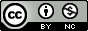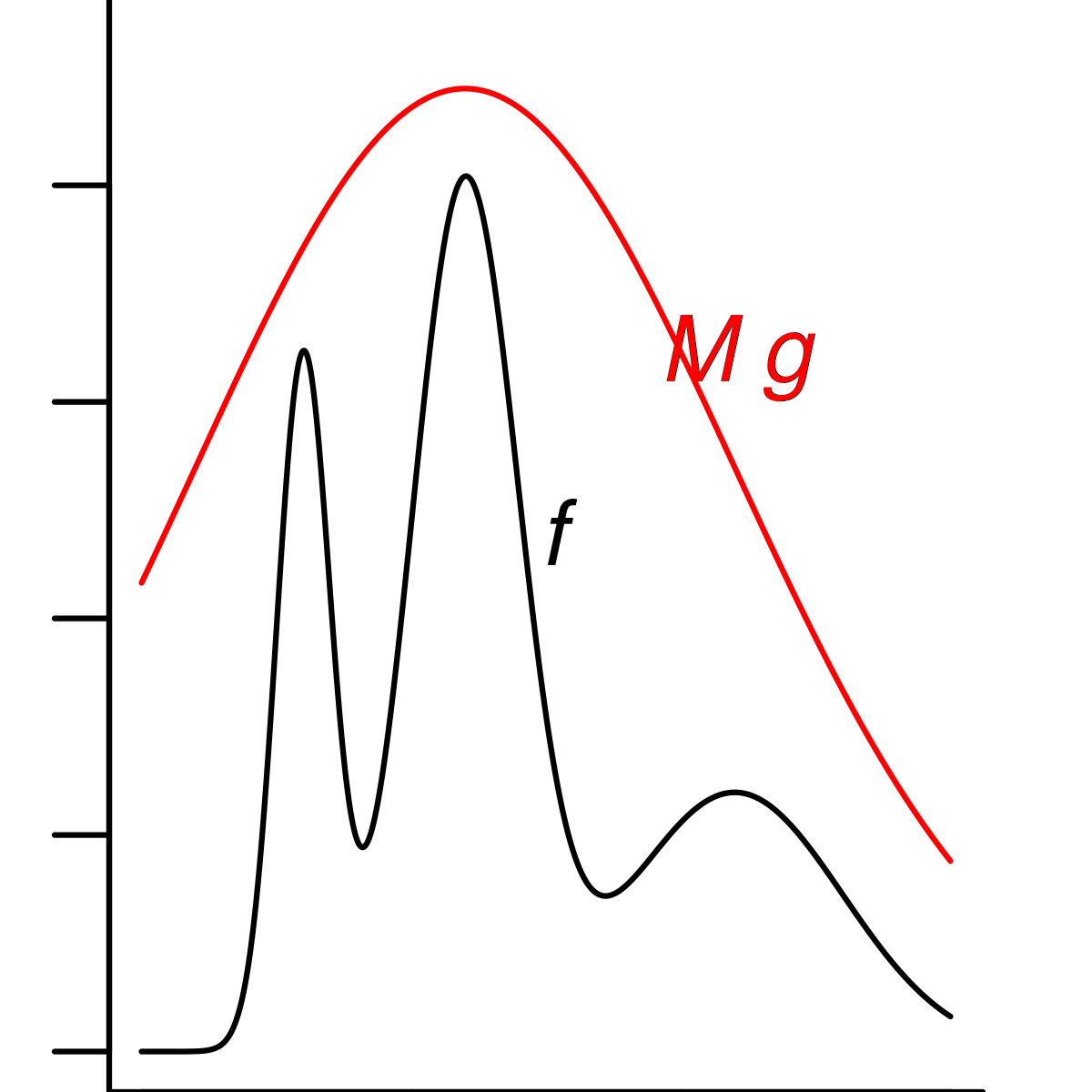Produced with R version 3.6.1.

## Objectives

1. To review some basic ideas in Monte Carlo computation and simulating random variables.

2. To provide a basic introduction to the Monte Carlo approach, and the generation of simulated random variables, for those who haven’t seen it before.

## Our context: Monte Carlo methods for POMP models.

Let’s consider a general POMP model. As before, let $$y_{1:N}^*$$ be the data, and let the model consist of a latent process $$X_{0:N}$$ and an observable process $$Y_{1:N}$$. Then the likelihood function is $\begin{eqnarray} \mathscr{L}(\theta) &=& f_{Y_{1:N}}(y_{1:N}^*\, ; \,\theta) \\ &=&\int_{x_0}\cdots\int_{x_N}\! f_{X_0}(x_0\, ; \,\theta)\prod_{n=1}^{N}\!f_{Y_n|X_n}(y_n^*{\, | \,}x_n\, ; \,\theta)\, f_{X_n|X_{n-1}}(x_n|x_{n-1}\, ; \,\theta)\, dx_0\dots dx_N. \end{eqnarray}$ i.e., computation of the likelihood requires integrating (or summing, for a discrete model) over all possible values of the unobserved latent process at each time point. This is very hard to do, in general.

Let’s review, and/or learn, some Monte Carlo approaches for evaluating this and other difficult integrals. An excellent technical reference on Monte Carlo techniques is Robert and Casella (2004).

## The fundamental theorem of Monte Carlo integration

• The basic insight of Monte Carlo methods is that we can get a numerical approximation to a challenging integral, $H = \int h(x)\, f(x)\, dx,$ if we can simulate (i.e., generate random draws) from the distribution with probability density function $$f$$.

• This insight is known as the fundamental theorem of Monte Carlo integration.

Theorem. Let $$f(x)$$ be the probability distribution function for a random variable $$X$$, and let $$X_{1:J}=\{X_j, j=1,\dots,J\}$$ be an independent and identically distributed sample of size $$J$$ from $$f$$. Let $${H_J}$$ be the sample average of $$h(X_1)\dots,h(X_J)$$, ${H_J} = \frac{1}{J}\,\sum_{j=1}^{J}\!h(X_j).$ Then $${H_J}$$ converges to $$H$$ as $$J\to\infty$$ with probability 1. Less formally, we write ${H_J} \approx \int\!h(x)\,f(x)\,dx.$

Proof. This is the strong law of large numbers, together with the identity that $\mathbb{E}[h(X)] = \int\!h(x)\,f(x)\,dx.$

• We can estimate the error in this approximation, because the empirical variance $V_J= \frac{1}{J-1}\,\sum_{j=1}^{J}\!\big[h(X_j)-H_J\big]^2$ approximates the true variance, $$\mathrm{Var}[h(X)]=\mathbb{E}\big[\big(h(X)-\mathbb{E}[h(X)]\big)^2\big]$$.

• The standard error on the approximation $$H_{J}\approx \mathbb{E}[h(X)]$$ is therefore $\sqrt{\frac{V_J}{J}}.$

• From the central limit theorem, the error is approximately normally distributed: $H_J-\mathbb{E}[h(X)]\;\sim\;\mathrm{normal}\left(0,\frac{V_J}{J}\right).$

• The fundamental theorem of Monte Carlo inspires us to give further thought on how to simulate from a desired density function $$f$$, which may itself be a challenging problem.

• We will review simulation, but first let’s consider a useful generalization of the fundamental theorem.

## Importance sampling

• Sometimes it is difficult to sample directly from the distribution of $$X$$.

• In this case, we can often make use of importance sampling, in which we generate random samples from another distribution (easier to simulate) and make the appropriate correction.

• Specifically, suppose we wish to compute $$\mathbb{E}[h(X)]$$, where $$X\sim{f}$$, but it is difficult or impossible to draw random samples from $$f$$.

• Suppose $$g$$ is a probability distribution from which it’s relatively easy to draw samples and let $$Y_{1:J}$$ be i.i.d. random variables drawn from $$g$$.

• Notice that $\mathbb{E}[h(X)] = \int\!h(x)\,f(x)\,\mathrm{d}x = \int\!h(x)\,\frac{f(x)}{g(x)}\,g(x)\, dx.$

• So, we can generalize the Monte Carlo integration theorem to give the Monte Carlo importance sampling theorem, $\mathbb{E}[h(X)] \approx \frac{1}{J}\,\sum_{j=1}^{J}\!h(Y_j)\,\frac{f(Y_j)}{g(Y_j)}.$

• We call $$w_j=f(Y_j)/g(Y_j)$$ the importance weights, and then we can write $\mathbb{E}[h(X)] \approx \frac{1}{J}\,\sum_{j=1}^{J} w_j\, h(Y_j).$

• Since $$\mathbb{E}[w_j] = \mathbb{E}[f(Y)/g(Y)]=1$$, we can modify this formula to give a self-normalized importance sampling estimate, $\mathbb{E}[h(X)] \approx \frac{\sum\!w_j\,h(Y_j)}{\sum\!w_j}.$

• The self-normalized estimate requires computation of $$w_j$$ only up to a constant of proportionality.

• The Monte Carlo variance associated with this estimate is $\frac{\sum\!w_j\,(h(Y_j)-\overline{h})^2}{\sum\!w_j}.$

• Obtaining accurate estimates requires some thought to the importance distribution $$g$$. Specifically, if the tails of $$g$$ are lighter than those of $$f$$, the Monte Carlo variance will be inflated and the estimates can be unusable.

## Simulation techniques for general distributions

• Simulation refers to the generation of random variables.

• The general problem of simulation is: given a probability distribution $$f$$, find a procedure that generates random draws from $$f$$.

• This is a very important problem in scientific computing and much thought and effort has gone into producing reliable simulators for many basic random variables.

• There are two basic ways of solving this problem:

1. The transformation method,

2. The rejection method.

### The transformation method

• This method works for discrete or continuous scalar random variables.

• Let $$f$$ be the probability distribution function we seek to draw from (known as the target distribution) and $$F$$ be the corresponding cumulative distribution function, i.e., $$F(x) = \int_{-\infty}^x f(v)\, dv$$.

• Let $$F^{-1}(u) = \inf\{x: F(x)\,\ge\,u\}$$ be the inverse of $$F$$.

• A basic fact is that, if $$X\!\sim\!f$$, then $$F(X)\!\sim\!\mathrm{uniform}(0,1)$$.
Proof: If $$f(X)>0$$, then $\begin{eqnarray} \mathbb{P}[F(X)\,\le\,u] &=& \mathbb{P}[X\,<\,F^{-1}(u)] \\ &=& F\big(F^{-1}(u)\big) = u. \end{eqnarray}$

• This suggests that, if we can compute $$F^{-1}$$, we use the following algorithm to generate $$X\!\sim\!f$$:

1. draw $$U \sim \mathrm{uniform}(0,1)$$.

2. let $$X = F^{-1}(U)$$.

### The rejection method

• The transformation method is very efficient in that we are guaranteed to obtain a valid $$X$$ from the density $$f$$ for every $$U \sim \mathrm{uniform}(0,1)$$ we generate.

• Sometimes, however, we cannot compute the inverse of the cumulative distribution function, as required by the transformation method.

• Under such circumstances, the rejection method offers a less efficient, but more flexible, alternative.

• We’ll see how and why this method works.

#### The rejection method for uniform random variables on arbitrary sets

• Let a random variable $$X$$ take values in $$\mathbb{R}^{\mathrm{dim}(X)}$$.

• Suppose that $$X$$ is uniformly distributed over a region $$D\subset \mathbb{R}^{\mathrm{dim}(X)}$$. This means that, for any $${A}\subset{D}$$, $\mathbb{P}[X\in{A]}=\frac{\mathrm{area}(A)}{\mathrm{area}(D)}.$ We write $X \sim \mathrm{uniform}(D).$

• Let’s suppose that we wish to simulate $$X\!\sim\!\mathrm{uniform}(D)$$.

• Suppose that we don’t know how to directly simulate a random draw from $$D$$, but we know $$D$$ is a subset of some nicer region $$U\subset \mathbb{R}^{\mathrm{dim}(X)}$$.

• If we know how to generate $$Y\!\sim\!\mathrm{uniform}(U)$$, then we can simply do so until $${Y}\in{D}$$, at which point we take $$X=Y$$.

• Since for any $$A\subset{D}$$, $\begin{eqnarray} \mathbb{P}[X\in A] &=& \mathbb{P}[Y\in A |Y\in D] \\ &=& \frac{\mathrm{area}(A)}{\mathrm{area}(U)}\Big{/}\frac{\mathrm{area}(D)}{\mathrm{area}(U)} \\ &=& \frac{\mathrm{area}(A)}{\mathrm{area}(D)}, \end{eqnarray}$ it follows that $$Y\!\sim\!\mathrm{uniform}(D)$$.

• Consider an analogy to throwing darts. If the darts are thrown in such a way as to be equally likely to land anywhere in $$U$$, then those that do land in $$D$$ are equally likely to land anywhere in $$D$$.#### The rejection method for dominated densities

• A useful little fact allows us to extend the rejection method from uniform distributions to arbitrary densities.

• Robert and Casella (2004) refer to this fact the fundamental theorem of simulation.

• Let $$h$$ be an arbitary positive, integrable function.

• Define $D=\{(x,u): 0{\le}u{\le}h(x)\},$ i.e., $$D$$ is the graph of $$h$$.

• Consider the random pair $$(X,U)\!\sim\!\mathrm{uniform}(D)$$.

• What is the marginal distribution of $$X$$? $\int_0^{h(x)}\!\mathrm{d}u = h(x)$

• So $$h$$ is the probability distribution function for $$X$$!

• To carry out the rejection method, simulating from $$g$$ to obtain a sample from $$f$$, we take our region $$D$$ to be the graph of $$Mg$$, i.e., $D = \{(x,y): 0\le y\le Mg(x),$ where $$M$$ is a constant chosen so that $$Mg(x)\le f(x)$$ for all $$x$$.

• We propose points $$(X,Y)$$ by drawing them uniformly from the area under the graph of $$M\,g$$.

• We accept the point $$(X,Y)$$ if it lies under the graph of $$f$$.

• Then, the $$X$$-component of the $$(X,Y)$$ pair is distributed according to $$f$$.

• This suggests the following rejection method for simulating an arbitrary random variable.

• Let $$f$$ be the target distribution and $$g$$ be another distribution function (from which it is easy to simulate) (see Figure below).

• Let $$M$$ be such that $$M\,g(x){\ge}f(x)$$ for all $$x$$.

• The following procedure simulates $$X\!\sim\!f$$.

1. draw $$Y\!\sim\!g$$ and $$U\!\sim\!\mathrm{uniform}(0,M\,g(Y))$$.

2. if $$U{\le}f(Y)$$, then let $$X=Y$$ else repeat step 1.Back